SLVSD45 December   2015

PRODUCTION DATA.

1. Features
2. Applications
3. Description
4. Revision History
5. Description (continued)
6. Pin Configuration and Functions
7. Specifications
8. Detailed Description
1. 8.1 Overview
2. 8.2 Functional Block Diagram
3. 8.3 Feature Description
4. 8.4 Device Functional Modes
9. Application and Implementation
1. 9.1 Application Information
2. 9.2 Typical Application
1. 9.2.1 Design Requirements
2. 9.2.2 Detailed Design Procedure
3. 9.2.3 Application Curve
10. 10Power Supply Recommendations
11. 11Layout
12. 12Device and Documentation Support
13. 13Mechanical, Packaging, and Orderable Information

• HKH|20
• KGD|0

## 9 Application and Implementation

NOTE

Information in the following applications sections is not part of the TI component specification, and TI does not warrant its accuracy or completeness. TI’s customers are responsible for determining suitability of components for their purposes. Customers should validate and test their design implementation to confirm system functionality.

### 9.1 Application Information

The TPS50601-SP device is a highly-integrated synchronous step-down DC-DC converter. The device is used to convert a higher DC-DC input voltage to a lower DC output voltage with a maximum output current of 6 A.

The TPS50601-SP user's guide is available on the TI website, SLVU499. The guide highlights standard EVM test results, schematic, and BOM for reference. (Basic design equations in following sections are provided for reference only)

### 9.2 Typical Application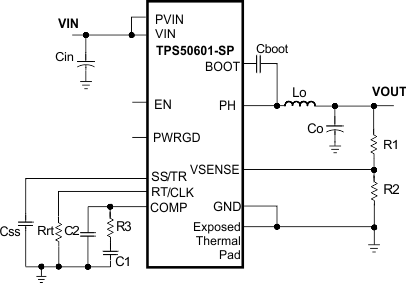Figure 29. Typical Application Schematic

#### 9.2.1 Design Requirements

This example details the design of a high frequency switching regulator design using ceramic output capacitors. A few parameters must be known in order to start the design process. These parameters are typically determined at the system level. For this example, we start with the following known parameters:

### Table 2. Design Parameters

DESIGN PARAMETER EXAMPLE VALUE
Output voltage 3.3 V
Output current 6 A
Transient response 1-A load step ΔVout = 5%
Input voltage 5 V nominal, 4.5 to 6.3 V
Output voltage ripple 33 mV p-p
Start input voltage (rising Vin) 4.425V
Stop input voltage (falling Vin) 4.234V
Switching frequency 480 kHz

#### 9.2.2.1 Operating Frequency

The first step is to decide on a switching frequency for the regulator. There is a trade off between higher and lower switching frequencies. Higher switching frequencies may produce smaller a solution size using lower valued inductors and smaller output capacitors compared to a power supply that switches at a lower frequency. However, the higher switching frequency causes extra switching losses, which hurt the converter’s efficiency and thermal performance. In this design, a moderate switching frequency of 480 kHz is selected to achieve both a small solution size and a high efficiency operation.

#### 9.2.2.2 Output Inductor Selection

To calculate the value of the output inductor, use Equation 20. KIND is a coefficient that represents the amount of inductor ripple current relative to the maximum output current. The inductor ripple current is filtered by the output capacitor. Therefore, choosing high inductor ripple currents impact the selection of the output capacitor since the output capacitor must have a ripple current rating equal to or greater than the inductor ripple current. In general, the inductor ripple value is at the discretion of the designer; however, KIND is normally from 0.1 to 0.4 for the majority of applications.

Equation 20.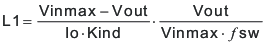For this design example, use KIND = 0.1 and the inductor value is calculated to be 2.78 µH. For this design, a nearest standard value was chosen: 3.3 µH. For the output filter inductor, it is important that the RMS current and saturation current ratings not be exceeded. The RMS and peak inductor current can be found from Equation 22 and Equation 23.

Equation 21.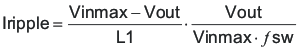Equation 22.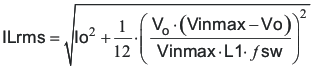Equation 23.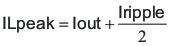For this design, the RMS inductor current is 6.02 A and the peak inductor current is 6.84 A. The chosen inductor is a Coilcraft MSS1048 series 3.3 µH. It has a saturation current rating of 7.38 A and a RMS current rating of 7.22 A.

The current flowing through the inductor is the inductor ripple current plus the output current. During power up, faults or transient load conditions, the inductor current can increase above the calculated peak inductor current level calculated above. In transient conditions, the inductor current can increase up to the switch current limit of the device. For this reason, the most conservative approach is to specify an inductor with a saturation current rating equal to or greater than the switch current limit rather than the peak inductor current.

#### 9.2.2.3 Output Capacitor Selection

There are three primary considerations for selecting the value of the output capacitor. The output capacitor determines the modulator pole, the output voltage ripple, and how the regulator responds to a large change in load current. The output capacitance needs to be selected based on the more stringent of these three criteria

The desired response to a large change in the load current is the first criteria. The output capacitor needs to supply the load with current when the regulator can not. This situation would occur if there are desired hold-up times for the regulator where the output capacitor must hold the output voltage above a certain level for a specified amount of time after the input power is removed. The regulator is also temporarily not able to supply sufficient output current if there is a large, fast increase in the current needs of the load such as a transition from no load to full load. The regulator usually needs two or more clock cycles for the control loop to see the change in load current and output voltage and adjust the duty cycle to react to the change. The output capacitor must be sized to supply the extra current to the load until the control loop responds to the load change. The output capacitance must be large enough to supply the difference in current for 2 clock cycles while only allowing a tolerable amount of droop in the output voltage. Equation 24 shows the minimum output capacitance necessary to accomplish this.

Equation 24.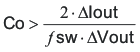Where ΔIout is the change in output current, Fsw is the regulators switching frequency and ΔVout is the allowable change in the output voltage. For this example, the transient load response is specified as a 5% change in Vout for a load step of 1A. For this example, ΔIout = 1.0 A and ΔVout = 0.05 x 3.3 = 0.165 V. Using these numbers gives a minimum capacitance of 25 μF. This value does not take the ESR of the output capacitor into account in the output voltage change. For ceramic capacitors, the ESR is usually small enough to ignore in this calculation.

Equation 25 calculates the minimum output capacitance needed to meet the output voltage ripple specification. Where fsw is the switching frequency, Vripple is the maximum allowable output voltage ripple, and Iripple is the inductor ripple current. In this case, the maximum output voltage ripple is 33mV. Under this requirement, Equation 25 yields 13.2 µF.

Equation 25.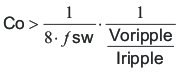Equation 26 calculates the maximum ESR an output capacitor can have to meet the output voltage ripple specification. Equation 26 indicates the ESR should be less than 19.7 mΩ. In this case, the ceramic caps’ ESR is much smaller than 19.7 mΩ.

Equation 26.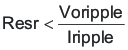Additional capacitance de-ratings for aging, temperature and DC bias should be factored in which increases this minimum value. For this example, a 47 μF 6.3V X5R ceramic capacitor with 3 mΩ of ESR is be used. Capacitors generally have limits to the amount of ripple current they can handle without failing or producing excess heat. An output capacitor that can support the inductor ripple current must be specified. Some capacitor data sheets specify the RMS (Root Mean Square) value of the maximum ripple current. Equation 27 can be used to calculate the RMS ripple current the output capacitor needs to support. For this application, Equation 27 yields 485mA.

Equation 27.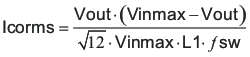#### 9.2.2.4 Input Capacitor Selection

The TPS50601-SP requires a high quality ceramic, type X5R or X7R, input decoupling capacitor of at least 4.7 µF of effective capacitance on the PVIN input voltage pins and 4.7 µF on the Vin input voltage pin. In some applications additional bulk capacitance may also be required for the PVIN input. The effective capacitance includes any DC bias effects. The voltage rating of the input capacitor must be greater than the maximum input voltage. The capacitor must also have a ripple current rating greater than the maximum input current ripple of the TPS50601-SP. The input ripple current can be calculated using Equation 28.

Equation 28.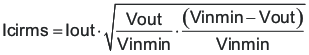The value of a ceramic capacitor varies significantly over temperature and the amount of DC bias applied to the capacitor. The capacitance variations due to temperature can be minimized by selecting a dielectric material that is stable over temperature. X5R and X7R ceramic dielectrics are usually selected for power regulator capacitors because they have a high capacitance to volume ratio and are fairly stable over temperature. The output capacitor must also be selected with the DC bias taken into account. The capacitance value of a capacitor decreases as the DC bias across a capacitor increases. For this example design, a ceramic capacitor with at least a 25-V voltage rating is required to support the maximum input voltage. For this example, one 10 μF and one 4.7-µF 25-V capacitors in parallel have been selected as the VIN and PVIN inputs are tied together so the TPS50601-SP may operate from a single supply. The input capacitance value determines the input ripple voltage of the regulator. The input voltage ripple can be calculated using Equation 29. Using the design example values, Ioutmax = 6 A, Cin = 14.7 μF, FSW = 480 kHz, yields an input voltage ripple of 213 mV and a RMS input ripple current of 2.95 A.

Equation 29.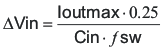#### 9.2.2.5 Slow Start Capacitor Selection

The slow start capacitor determines the minimum amount of time it takes for the output voltage to reach its nominal programmed value during power up. This is useful if a load requires a controlled voltage slew rate. This is also used if the output capacitance is very large and would require large amounts of current to quickly charge the capacitor to the output voltage level. The large currents necessary to charge the capacitor may make the TPS50601-SP reach the current limit or excessive current draw from the input power supply may cause the input voltage rail to sag. Limiting the output voltage slew rate solves both of these problems. The soft start capacitor value can be calculated using Equation 30. For the example circuit, the soft start time is not too critical since the output capacitor value is 47 μF which does not require much current to charge to 3.3 V. The example circuit has the soft start time set to an arbitrary value of 3.5 ms which requires a 10-nF capacitor. In TPS50601-SP, Iss is 2.5 µA typical, and Vref is 0.795 V.

Equation 30.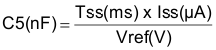#### 9.2.2.6 Bootstrap Capacitor Selection

A 0.1-µF ceramic capacitor must be connected between the BOOT to PH pin for proper operation. TI recommends to use a ceramic capacitor with X5R or better grade dielectric. The capacitor should have a voltage rating of 10 V or higher.

#### 9.2.2.7 Undervoltage Lockout (UVLO) Set Point

The UVLO can be adjusted using the external voltage divider network of R6a and R7a. R6a is connected between VIN and the EN pin of the TPS50601-SP and R7a is connected between EN and GND . The UVLO has two thresholds, one for power up when the input voltage is rising and one for power down or brown outs when the input voltage is falling. For the example design, the supply should turn on and start switching once the input voltage increases above selected voltage (UVLO start or enable). After the regulator starts switching, it should continue to do so until the input voltage falls below (UVLO stop or disable) voltage. Equation 4 and Equation 5 can be used to calculate the values for the upper and lower resistor values. For the stop voltages specified the nearest standard resistor value for R6a is 10.0 kΩ and for R7a is 3.4 kΩ.

#### 9.2.2.8 Output Voltage Feedback Resistor Selection

The resistor divider network R5 and R6 is used to set the output voltage. For the example design, 10 kΩ was selected for R6. Using Equation 31, R5 is calculated as 31.25 kΩ. The nearest standard 1% resistor is 31.6 kΩ.

Equation 31.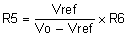#### 9.2.2.8.1 Minimum Output Voltage

Due to the internal design of the TPS50601-SP, there is a minimum output voltage limit for any given input voltage. The output voltage can never be lower than the internal voltage reference of 0.8 V. Above 0.8 V, the output voltage may be limited by the minimum controllable on time. The minimum output voltage in this case is given by Equation 32

spacer

Equation 32. VOUTmin = Ontimemin × ƒsmax (VINmax + IOUTmin (RDS2min - RDS1min)) - IOUTmin (RL + RDS2min)

where

• VOUTmin = Minimum achievable output voltage
• Ontimemin = Minimum controllable on-time (175 ns maximum)
• ƒsmax = Maximum switching frequency including tolerance
• VINmax = Maximum input voltage
• IOUTmin = Minimum load current
• RDS1min = Minimum high-side MOSFET on-resistance (36-32 mΩ typical)
• RDS2min = Minimum low-side MOSFET on-resistance (19 mΩ typical)
• RL = Series resistance of output inductor

#### 9.2.2.9 Compensation Component Selection

There are several industry techniques used to compensate DC/DC regulators. The method presented here is easy to calculate and yields high phase margins. For most conditions, the regulator has a phase margin between 60 and 90 degrees. The method presented here ignores the effects of the slope compensation that is internal to the TPS50601-SP. Since the slope compensation is ignored, the actual cross over frequency is usually lower than the cross over frequency used in the calculations. Use WEBENCH, Pspice model for simulation.

First, the modulator pole, fpmod, and the esr zero, fzmod must be calculated using Equation 33 and Equation 34. For Cout, use a derated value of 22.4 µF. use Equation 35 and Equation 36 to estimate a starting point for the closed loop crossover frequency fco. Then the required compensation components may be derived. For this design example, fpmod is 12.9 kHz and fzmod is 2730 kHz. Equation 35 is the geometric mean of the modulator pole and the esr zero and Equation 36 is the geometric mean of the modulator pole and one half the switching frequency. Use a frequency near the lower of these two values as the intended crossover frequency fco. In this case Equation 35 yields 175 kHz and Equation 36 yields 55.7 kHz. The lower value is 55.7 kHz. A slightly higher frequency of 60.5 kHz is chosen as the intended crossover frequency.

Equation 33.Equation 34.Equation 35.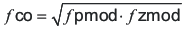Equation 36.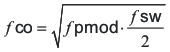Now the compensation components can be calculated. First calculate the value for R2 which sets the gain of the compensated network at the crossover frequency. Use Equation 37 to determine the value of R2.

Equation 37.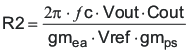Next calculate the value of C3. Together with R2, C3 places a compensation zero at the modulator pole frequency. Equation 38 to determine the value of C3.

Equation 38.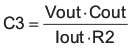Using Equation 37 and Equation 38 the standard values for R2 and C3 are 1.69 kΩ and 8200 pF.

An additional high frequency pole can be used if necessary by adding a capacitor in parallel with the series combination of R2 and C3. The pole frequency is given by Equation 39. This pole is not used in this design.

Equation 39.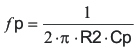#### 9.2.3 Application CurveA. Per EVM - for additional details see the User's Guide, SLVU499
Figure 30. Typical Switching Waveform for 100-kHz Switching Operation